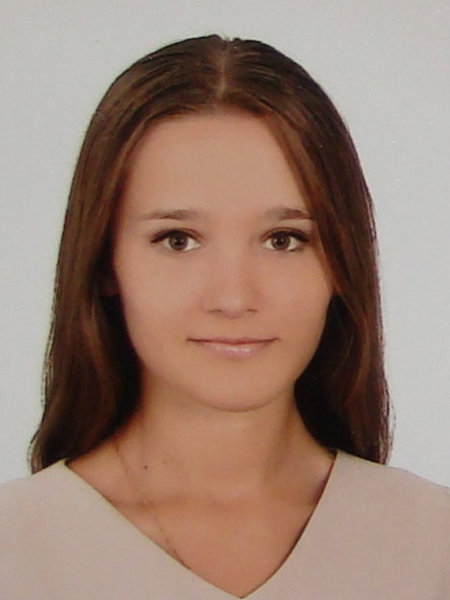Українською | In English

Elena V. Ovcharenko

Senior teacher of the departmentWorks in KPI since 2013

Teaches on faculties: RTF, FIOT

Courses taught:
Higher mathematics at the Faculty of Management and Marketing

Lectures schedule (in Ukrainian) на rozklad.kpi.ua

Education:

NTUU "KPI", 2008, Physical and Mathematical Faculty, Math. Mathematician. Teacher

Candidate of physical and mathematical sciences since 2013, 01.01.02 - differential equations, topic: "New generalizations of hypergeometric type functions and their applications"

Scientific interests:

The theory of special functions, differential and integral equations, integral transforms

Latest papers:

See all

8. Ovcharenko O. Differential formulas for q-integral representation of (τ, β) - generalized hypergeometric function / O. Ovcharenko // Scientific news of "KPI". - 2011. - № 4. - P. 71 - 74. (In Ukrainian)

9. Virchenko N.O. Some integral operators with the generalized hypergeometric function Gauss / N. Virchenko, O. Rumyantseva // Boundary value problems for differential equations. Collection. Science. etc. - Chernivtsi, 2008. - Issue. 17. - P. 234 - 239. (In Ukrainian)

10. Ovcharenko O. Some results of calculation of sums include (τ, β)-generalized hypergeometric function Gauss / O. Ovcharenko // Boundary value problems for differential equations. Collection. Science. etc. - Chernivtsi, 2009. - Issue. 18. - P. 266 - 275. (In Ukrainian)